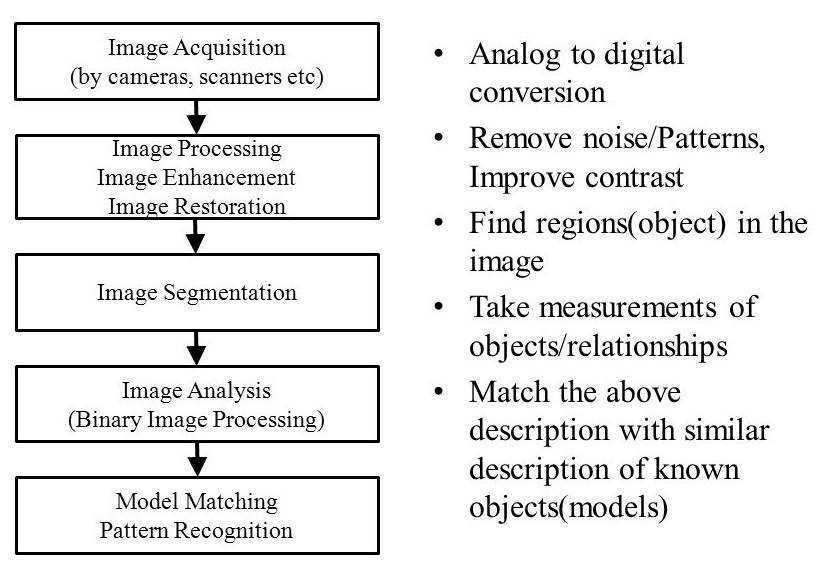### Notes of Artificial Intelligence [CT 653]

#### Applications of AI

Neural Networks Structure

Neutral networks and ANN:

- Neural network is the network of neurons as found in the brain.
- ANN is the network of artificial neurons so as to correspond the system approximately to the parts of brain.
- Artificial neurons are approximation of brain neurons, which can be physical device or mathematical constructs.
- ANN provides parallel computation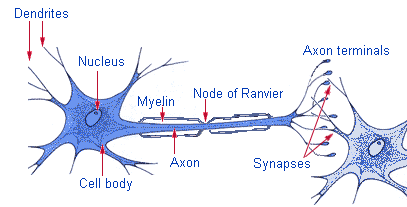Human brain and ANN

- As ANN is the approach to model real human brain, the knowledge of working principle of brain is very important
- Neurons encode activation or output as a series of electrical pulses.
- The cell body(soma) processes incoming activations and converts them into o/p activations
- The nucleus contains the genetic material
- Dendrites receive activation from other neurons
- Axon transmits o/p activation to other neurons
- The junction between axon and dendrite is called synapses.
- In ANN , the brain is modeled as
a) Neuron represents soma
b) Input represents dendrite
c) Output represents axon
d) Weight represent synapse
- The comparison between ANN and brain
1) Processing element -> synapse(brain)

Basic terminology

1. weighting factor(w)
The weighting factor is the value given to each i/p to determine its strength. The neuron computes the weighted sum of i/p and compares with threshold. If it is less, the neuron o/p is -1. If it is greater or equal, the neutron activates and outputs +1.

2. Threshold(w)
Threshold is the minimum value required by the node as input in order to activate the node

3. Activation function(f)
Activation function performs mathematical operation on the signal output.
Eg: for X = Summation from i = 1 to n [x(i) * w(i)]
Sign function)f=+1 ;if x≥ϴ
-1 ; if x <ϴ
(step function) f==+1 ;if x≥ϴ
; if x <ϴ
(sigmoid function)f=1/(1+e-x )linear function f=x

Basic Structure of Artificial Neuron: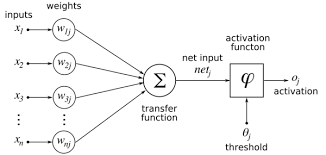Types of Neural Network:

1. Single Layer Feed Forward
2. Multi Layer Feed Forward
3. Recurrent or Feedback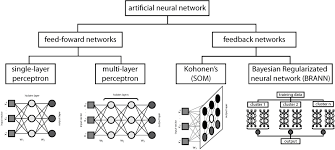- A solution to a problem without algorithm solution can be obtained.
- If one element fails, the system does not fail as it is overcome by other elements.
- Learning is efficient.
- Parallel processing makes the performance better.

Applications
a) financial modeling-> stock prediction
b) robotics -> automatic adaptable robot
d) Bata analysis
e) Prediction
f) Bioinformatics -> DNA sequencing

Learning process
1. Supervised learning
- Inferring a function from training data that consists of training examples. Eg: classification

2. Unsupervised learning
- Finding hidden structure in unlabeled data. There is no distinction between explanatory and dependent variables.
Eg: clustering

Learning rate (α)
- Learning rate is a constant that affects the speed of learning. Is affects the performance of algorithm

Adeline network is a neural network with following characteristics:
1) Inputs are +1 or -1;
2) Outputs are +1 or -1
3) Uses a bias input
4) Trained using delta rude / least mean squares
5) During training, activation function is identify function a
6) After training, activation is threshold function

Algorithm
1) Initialize weights to small random values and select k.
2) For each i/p vector s, with target o/p t , set i/p to s
3) Complete neuron inputs. Y_in =b+
4) Use delta rule to update bias and weights.
B(new) = b(old)+ α(t-y-in)
W;(new)=w;(old) +α(t-y-in)x;
5) Repeat until largest wt. change across all training samples is less than a specified tolerance.

Example: AND function (α = 0.1, tolerance = 0.1)

The activation function is :
Y= 1; Y_in >=0
-1 ; Y_in <0
Where, Y_in =b+

1. Initialization:
- Set weights to small random values
- Random values
X1 x2 bias t
1 1 1 1
1 -1 1 -1
-1 1 1 -1
-1 -1 1 -1

2. First cycle
#run 1[(1,1),1]
Y_in = 0.1+0.2*1+0.3*1=0.6
.·. b(new)=0.1 + 0.1(1-0.6) = 0.14
.·. w1=0.2+0.1(1-0.6)1=0.24
.·. w2=0.3+0.1(1-0.6)1=0.34
Largest wt. change =o.o4

#run 2 [(1,-1),-1]
Y_in=0.14+0.24*1+0.34*-1=0.04
.·.b=0.14+0.1(-1-0.04)1=0.04
.·.w1=0.24+0.1(-1-0.04)1=0.14
.·.w2=0.34+0.1(-1-0.04)-1=0.44
Largest wt. Change=0.1

#run 3[(-1,1),-1]
Y_in=0.34
.·.b=0.09
.·.w1=0.27
.·.w2=0.31
Largest wt. change =0.13

#run 4 [(-1,-1),-1]
Y_in =-0.67
B=-00.27
W1=0.43
W2=0.47
Largest wt. Change=0.16

3. Second cycle
#run1
Y_in =0.63
B=-0.233
W1=0.46
W2=0.50
Largest change=0.031

#run2
Y_in =-0.273
B=-0.30
W1=0.39
W2=0.57
Largest change=0.07

#run 3
Y_in =-0.12
B=-0.38
W1=0.47
W2=0.48
Largest change=0.09

#run 4
Y_in =-1.33
B=-0.34
W1=0.43
W2=0.44
Largest change=0.04

In second cycle, largest wt. Change across all training samples is less than 0.1
So, the solution is :
B=-0.34
W1=0.43
W2=0.448

Perceptron

Perceptron is a feed forward neural network in which weighted sun of i/p is applied to an activation function that produces o/p +q if i/p is positive and –q if i/p is negative . The connections are unidirectional . it can learn any linearly separable function.

Algorithm

1. Initialization
- Set initial weights w; and threshold ϴ to random numbers in range [-0.5,+0.5]
- If error e(p) is +ve , increase perceptron 0/p y(p) else decrese y(p) ep =yd-yact

2. Activation
- Activate perceptron by applyin inputs x;(p) and desired o/p yd(p) .calculate actual o/p at iteration p=1
Y(p)=step[Summantion i = 1 to n {xi(p) * wi(p)} - ϴ]

3. Weight training
- Update weights
W i=(p+1)=wi(p)+wi(p)
Where,i(p)=α-xi(p)-e(p)=weight correction

4. Iteration
- Increase p by 1 and repeat from step 2 until the process converges.

Example: Perceptron network to perform AND Gate ( α = 0.1, ϴ = 0.2)

Initialization
- Set w1=0.3 and w2 =-0.1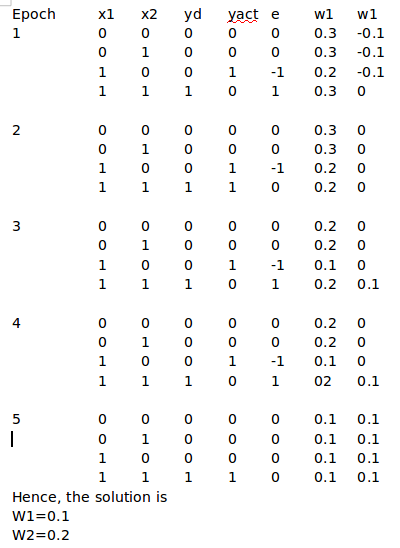Back Propagation Algorithm

Back propagation is the method of training ANN used in conjunction with optimization method.
It calculates the gradient of a loss function with respect to all the weights in the network the gradient is fed to optimization method which in turns uses it to update the weights to minimize the loss function
Back propagation algorithm is divided into two phases : propagation and wt. update

Algorithm:

Let A = no. of units in i/p layer
C=no. of units in o/p layer
B= no of units in hidden layer
Xi= activation level of units in i/p
Yi= in hidden layer
Oi =in o/p layer
W1ij=wt from i/p to hidden layer
W2ij=wt from hidden to o/p layer

1) Initialize weights randomly between -0.1 and 0.1
2) Initialize activations of thresholding units
X0=1.0 and h0=1.0
3) Choose i/p-o/p pair (say x; and y;)assign activation level to i/p units
4) Propagate activations from i/p layer to hidden layer using activation function
H(i)= 1/[1 + e ^ {- Summation i = 0 to A (w1(i)(j) * x1)}] --------> for j=1,………..b
5) Propagate activation from hiden layer to o/p layer using:
Oj= 1/[1 + e ^ {- Summation i = 0 to B (w2(i)(j) * h(i))}] -------------> for j=1,………..c
6) Compute error o funits in o/p layer (s2j)
δ2(j)=oj(1-oj)(yj-oj) for j=1……..c
7) Compute errors of units in hidden layer ()
δ1(j) = hj (1-hj) [Summation i = 1 to c {δ2(i) * w2(j)(i)}] for j= 1…….,b
8) Adjust weights between hidden layer and o/p layer
∆w2ij=αδ2j .hi
9) Adjust weights between i/p layer and hidden layer
∆wij=αδ2j .xi
10) Repeat from step 4 until converges

Hopfield Network

- Hopfield network is a network with memory
- The features are :-
a) Distributed representation
b) Asynchronous control
d) Fault tolerate

Steps involved

- Processing units are in aone of two sates:
Active or inactive.
- A +ve wt. indicates two units tend to activate each other .
- A –ve wt. indicates an active unit deactivates a neighboring unit

1) A random unit is chosen
2) If any of its neighbors are active ,the unit computes sum of weights on the connections to active neighbors
3) If sum is +ve , the unit becomes active
4) Otherwise , it becomes inactive
5) Repeat from step 1, until it reaches stable state.

Kohonen Network

- Unsupervised learning network based on concept of graded learning
- Feed forward network with two layers:
a) i/p layer
b) b)o/p or kohonen layer
- Every neuron of i/p layer is connected to every neuron of kohonen layer.
- Each connection is associated with weight wij

Algorithm:

1) Initialize weights wij randomly
2) Initialize neighborhood and learning rate
3) For all input vector at random:
a) Select i/p vector at random
b) Find kohonen neuron j that has its associated wt. vector closest to input vector. Closeness can be measured by distance function.
Dist(wj,x)= for i=1 to n the one with least distance is chosed.
c) Modify weight of all neurons in neighborhood of radius r of selected neuron
Wj(t+1)=wj(t)+α[x(t)-wj(t)]
d) Update α by reducing it gradually.

Architecture of Expert System

An expert system is an intelligent program that solves problems in a narrow problem area by using expert, specific knowledge rather than an algorithm. If simulates the decision making process of a human expert in a specific domain

Features

a) Reasoning capacity
b) Cope with uncertainty
c) Use of knowledge not data
d) Symbolic knowledge representation
e) Use meta knowledge
f) Use user interface

a) Provides consistent answer for repetitive problem.
b) Maintains significant level of information.
c) Proper explanation of decision making ask question like human experts.

a) Lack of common sense
b) Unable to make creative response in unusual situation
c) Error in knowledge base leads to wrong decision
d) Unable to adopt to changing environment.

Human expert and expert system

Human expert
- Perishable
- Slow processing
- Unpredictable
- Slow reproduction
- Expensive
- Creative ability
- Common sense

Expert system
- Permanent
- Fast processing
- Consistent
- Quick replication
- Affordable
- Narrow focus
- No creativity
- Instruction
- Knowledge Base

Architecture of Expert System:

1. Knowledge base: it is a data structure which contains knowledge in the form of rules, generally an IF-THEN statement
2. Working memory : it is a data structure that contains problem specific knowledge
3. Inference engine: it is a set of procedures for matching knowledge base with problem specific working memory.
4. User interface: it controls the communication between user and system.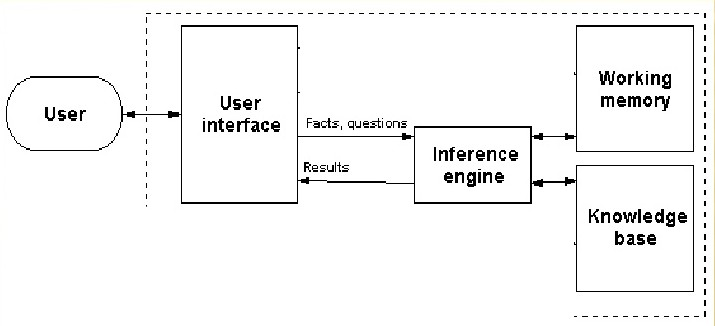Declarative and Procedural Knowledge

- Declarative knowledge means knowing what it contains surface level and descriptive representation of knowledge. It provides facts, about truth.
- Procedural knowledge means ‘knowing how’ it contains explanations

Development of Expert System

a) Knowledge acquisition
- It deals with how to acquire knowledge
- It involves finding knowledge through human experts and converting it into rules to form a knowledge base.

b) Knowledge representation
- It involves representation of knowledge in computer acceptable form.
- It may be logical or structured

c) Knowledge inferencing
- It involves acquiring new knowledge from existing facts using rule based reasoning

d) Knowledge transfer
- It involves organizing , capturing and distributing knowledge to ensure availabity for future users.

Example: MYCIN
Mycin is an expert system for treating blood infections. It diagnoses patients based on reported symptoms and test results. It recommends a course of treatment. It uses backward chaining for reasoning.

Natural Language Processing and Analysis Levels

Natural language processing is the process of analyzing of input provided in the form of written or spoken human language and convert it to a form understandable by the computer system. It involves processing of speech grammar and meaning.

Issues in NLP

1) ambiguous-> unclear in meaning
Eg: he is beating around the bush.
Direct: beats around the bush
Actual: wasting time

2)imprecise-> not exact
Eg: I am waiting for a long time.

3)Incomplete -> not complete
eg: come here

4) Inaccurate -> mistakes in spelling or pronunciation:

NLP steps

I. Input source
- The input for NLP system is text or speech
- Quality of input determines quality of output

II. Segmentation
- The input text is divided in segments, each of which is called frame.
- The frame is then analyzed.

III. Syntactic analysis
- Syntactic analysis produces the grammatical structure.
- It checks the syntax or grammar of input
- It helps in grouping of phrases
- It also consists of parse trees.
Eg: syntax:
<->
<->
<->
<->
<-> a l an l the
<-> man l dog
<-> likes l bites

“The man bites the dog”
Syntactic analysis:

IV. Semantic analysis
- It involves conversion of syntactic representations into a meaning representation.
- It performs word sense determination and sentence level analysis.
- Word sense determination determines meaning each word. This phase is difficult because a single word has different meaning in different contexts
- Sentence level analysis means assigning meaning to the sentence

V. Pragmatic analysis
- It involves aspects of meaning that depends upon facts about real world such as pronouns referring expressions, meaning of a set of propositions, etc.

Applications
a) machine translation
b) database access
c) text interpretation

Introduction to Machine Vision

Machine vision is the approach to make machine able to visualize the objects. The main goal is to create a model of real world from images.

The steps in machine vision are: